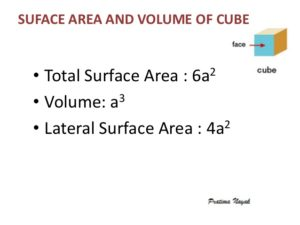# Curved Surface Area Of Cube : Java Program | 3 Simple Ways

How to write a java program to calculate the curved surface area of a cube? Here we cover the code in three different ways. Using standard values, command line arguments, method calling. Do check out, at the end of the codes; we also added an online execution tool such that you can execute each program individually. For more programs do check out here: Beginner Programs with outputs and Java interview programs for beginners here.

[table id=2 /]

Q: How to calculate the curved surface area of the cube?

A: Here, the Curved surface area is total area of the curved path. The math formula is:Image Source: SlideShare

Java Code Example – 1 ( Using Standard Values with Outputs )

Here we used standard values, ( 4 x a x a). You can use the below sample in all math oriented programs. Followed by command line arguments ( parse Java) and method calling method. At the end of the three programs, we do also embed an online execution tool, where you can execute the below codes.

Output ( For standard Values – 1 )

Code: 2 ( Using Command-Line Arguments )

Here we used command line arguments, here we share a complete guide on what are command line arguments in java with examples. Do check it out. In simple command line arguments are, displaying inputs through the console. Here is the sample program for the curved surface area of the cube.

Output: ( Using command line arguments )

Sample Program -3 ( Using method calling )

Here we used method calling, do check it out

Output: ( Using Return Invoke Method )

Soon, we are going to add an online execution tool, where you can execute each program. In case if you need any custom program for your project do contact us.

x

## Plus Star Pattern Java Program | Patterns

Java program to print plus star pattern program – We have written the below print/draw ...